# Continuous Statistical Distributions¶

## Overview¶

All distributions will have location (L) and Scale (S) parameters along with any shape parameters needed, the names for the shape parameters will vary. Standard form for the distributions will be given where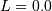and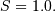The nonstandard forms can be obtained for the various functions using (note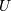is a standard uniform random variate).

Function Name Standard Function Transformation
Cumulative Distribution Function (CDF)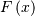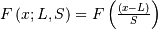Probability Density Function (PDF)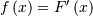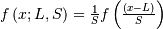Percent Point Function (PPF)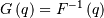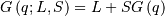Probability Sparsity Function (PSF)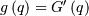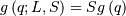Hazard Function (HF)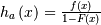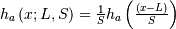Cumulative Hazard Functon (CHF)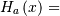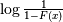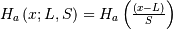Survival Function (SF)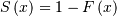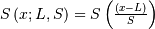Inverse Survival Function (ISF)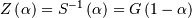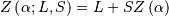Moment Generating Function (MGF)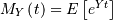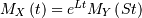Random Variates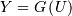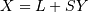(Differential) Entropy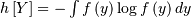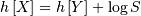(Non-central) Moments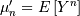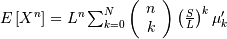Central Moments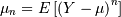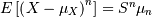mean (mode, median), var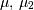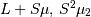skewness, kurtosis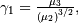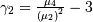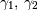### Moments¶

Non-central moments are defined using the PDF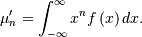Note, that these can always be computed using the PPF. Substitute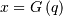in the above equation and get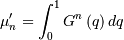which may be easier to compute numerically. Note that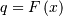so that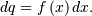Central moments are computed similarly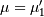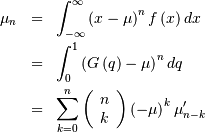In particular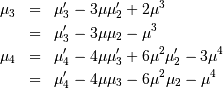Skewness is defined as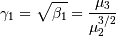while (Fisher) kurtosis is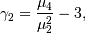so that a normal distribution has a kurtosis of zero.

### Median and mode¶

The median,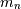is defined as the point at which half of the density is on one side and half on the other. In other words,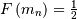so that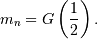In addition, the mode,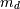, is defined as the value for which the probability density function reaches it’s peak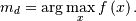### Fitting data¶

To fit data to a distribution, maximizing the likelihood function is common. Alternatively, some distributions have well-known minimum variance unbiased estimators. These will be chosen by default, but the likelihood function will always be available for minimizing.

If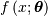is the PDF of a random-variable where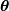is a vector of parameters ( e.g.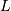and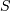), then for a collection of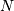independent samples from this distribution, the joint distribution the random vector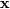is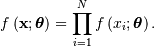The maximum likelihood estimate of the parametersare the parameters which maximize this function withfixed and given by the data: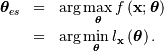Where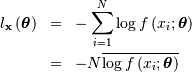Note that ifincludes only shape parameters, the location and scale-parameters can be fit by replacing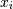with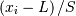in the log-likelihood function adding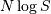and minimizing, thus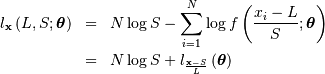If desired, sample estimates forand(not necessarily maximum likelihood estimates) can be obtained from samples estimates of the mean and variance using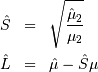where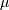and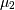are assumed known as the mean and variance of the untransformed distribution (when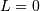and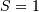) and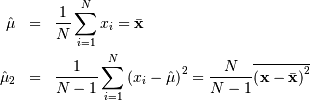### Standard notation for mean¶

We will use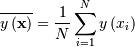whereshould be clear from context as the number of samples